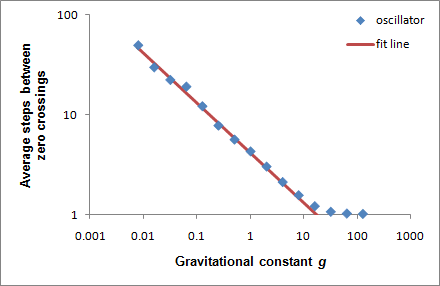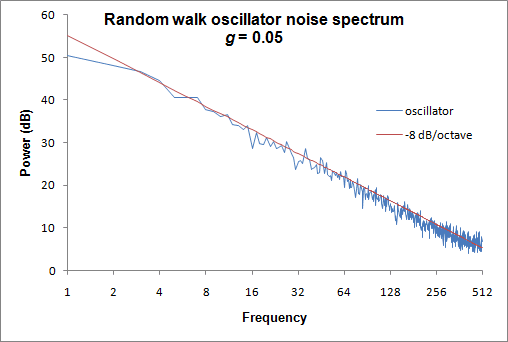### Random walk oscillator

May 2017: This is from my other blog that's no longer online. The original comments are no longer available, but you are welcome to add more.

Here's an experiment. Suppose I want to make a random walk that I can control, that doesn't wander aimlessly all over, but rather is attracted to zero. The attraction back to zero wouldn't act like a force that causes steps away from zero to become smaller in size; rather, steps toward zero are simply more probable further away from zero, regardless of the step size. I'll invent a random walk that does this.

We generate random walk steps in a generalized way via some inverse cumulative distribution function (cdf), which could represent a gaussian, a black swan, binary ±1 steps, whatever. This inverse cdf, in turn, takes as an input a random probability p that's uniformly-distributed between 0 and 1. (If you want uniformly-distributed steps, the step size simply equals the input p, or a multiple of p.)

We need to skew this uniformly-distributed input so that the resulting distribution of probable steps is biased toward zero. That is, when the random walk is near zero, the input probabilities distribute uniformly. The further away the random walk gets from zero, the more strongly the input distribution of p is adjusted to give higher probability to steps that return the random walk to zero.

I came up with an expression that bends the uniform cumulative distribution according to distance from zero: $$A(x) = \frac{ e^{-dgx} - 1} {e^{-dg}-1}, \quad 0\le x \le 1,\, g>0$$

A(x) here means "adjusted cumulative uniform distribution function". d is the current distance, in positive or negative standard deviations, that the random walk has wandered from zero. g is a "gravitational constant" greater than 0, which controls how strongly the random walk is biased toward zero.

At d=0, this equation looks like it would either go to zero (due to zero in the numerator) or blow up (due to zero in the denominator). Actually, according to L'Hôpital's rule, A(x)→x as d→0.  When d=0, the equation becomes a uniform cumulative distribution, a 45° line going from (0,0) to (1,1) as shown below, generating values uniformly across the interval [0,1]. This plot uses g=1:The probability density function (pdf) is the derivative of the cumulative density function (cdf) above: $$\alpha(x) = - \frac{dg\, e^{-dgx}} {e^{-dg}-1}$$

Again, at d=0, L'Hôpital's rule tells us that α(x)→1 as d→0; therefore, the pdf at d=0σ corresponds to the flat uniform distribution as shown below. You can see that when d>0 (that is, the random walk has wandered above zero), the pdf has more weight to the left, and when d<0 it's heavier to the right.To generate random values from these altered uniform distribution, we just need the inverse of the cdf: $$A^{-1}(p) = - \frac {\ln(p (e^{-dg}-1)+1)} {dg}$$ ...where p is a uniformly distributed random probability between 0 and 1. Again, from L'Hôpital's rule, A−1(p)→p as d→0.

Let's try it with some different distributions to generate random steps. The uniform distribution centered around zero presents a simple case. The cdf and its inverse are: $$U(x;\mu,\sigma)= \begin{cases} 0 & \text{for }x < -\sigma\sqrt{3} \\ \frac{1}{2} \left( \frac{x-\mu}{\sigma \sqrt{3}} +1 \right) & \mbox{for }\sigma\sqrt{3} \le x < \sigma\sqrt{3} \\ 1 & \mbox{for }x \ge \sigma\sqrt{3} \end{cases}$$ $$U^{-1}(p;\mu,\sigma) = \sigma\sqrt{3}(2p-1) +\mu\,\, \mbox{ for }0 \le p \le 1$$

To generate random values, we set μ=0 and plug in random probabilities generated by A−1 in place of p inside U−1. Each new value is added to the previous value to generate the random walk oscillator. Here's how it looks using the gravitational constant g=1:Now let's use the normal distribution. We generate steps using the inverse cumulative normal distribution function N−1(p;μ,σ), again replacing the probability p with the output of A−1. As before, the gravitational constant g=1. The results look similar:In fact, the results are similar. Much empirical observation reveals that regardless of the distribution used:

• The standard deviation determines the overall amplitude of the oscillator.
• Typically the amplitude extends 3 to 4 standard deviations on either side of zero, although theoretically the amplitude is unbounded and unconstrained.
• The standard deviation of the distribution steps adjusted by A−1 is about the same as the unadjusted distribution steps.
• The gravitational constant g determines the average duration between zero crossings.

That last item excited me. It means I can come up with a rule for the average wavelength of the oscillator. It means I can include this oscillator in other experiments in a controlled but non-periodic way.

So I generated a few thousand steps for my random walk oscillator, using the uniform distribution, the normal distribution, and a binary distribution. I plotted the average number of steps between zero crossings as a function of g. In each case I got the same result. Plotted on a double-log scale, the relationship can be modeled by a line.As g gets large, the zero crossings become 1 step apart. The bend in the data at the right occurs because 1 step is the smallest increment possible. This line fits best: $$\ln z = \sqrt 2 - \frac{1}{2} \ln g$$

For any desired average steps z between zero crossings: $$g = \frac {e^{2 \sqrt 2}}{z^2}$$

Testing this, I took my oscillator based on N−1, divided the standard deviation by 10, multiplied the number of steps by 10, and calculated a new g based on 10 times the original average number of steps between zero crossings. It looked like this:Although it looks a bit different, I verified that the range is still 3 or 4 standard deviations from zero, and the average steps between zero crossings are approximately 10 times what I had before. Everything works fine.

Now that I have an oscillator that produces a waveform around zero, I wonder what it sounds like? That is, what does its power spectrum look like? To make a power spectrum, I generated 8,192 values at g=0.05, and calculated a Fast Fourier Transform (FFT) power spectrum of 1,024 values at a time, sliding this window 128 steps 128 times, and also removed any drift and DC offset from the 1,024 input values prior to calculating the FFT. I averaged the power of the resulting 128 FFTs and plotted them on a decibel scale:The result is a noise spectrum that falls off at about −8 dB per octave. This is steeper than brownian noise, which falls off at −6 dB per octave, which in turn is steeper than the 1–3 db/octave falloff of pink noise. Brownian noise sounds like a low roar resembling a waterfall (listen to a sample). My random walk oscillator would sound like a somewhat lower roar, being heavier in the low frequencies.